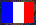# Analysis of the Debian Bug 153548 in glibc

Note: This bug was fixed in the glibc CVS on 2003-01-20.

When testing the GNU C library (glibc) with worst cases for correct rounding, I found five (completely) incorrect values on my PowerPC machine for the cosine function, amongst 1576 worst cases. Usually, when there is a bug, the number of failures is much larger. So, why only five?

First, let us recall the incorrect values. As I said in my bug report, the problem occurs in a small interval (around 0.80 to 0.83) only:

```Error: cos (line 33)
[arg.]  x = 0.80190127184058835
[corr]  y = 0.69534156199418473
[libm]  z = 0.71867942238767868
Error: cos (line 34)
[arg.]  x = 0.8107741500588278
[corr]  y = 0.68893751943804493
[libm]  z = 0.72482073253360613
Error: cos (line 35)
[arg.]  x = 0.81808070960270574
[corr]  y = 0.68362323105565981
[libm]  z = 0.72983510326718326
Error: cos (line 36)
[arg.]  x = 0.81909979079003903
[corr]  y = 0.6828791149804222
[libm]  z = 0.73053139174408888
Error: cos (line 37)
[arg.]  x = 0.82926610298753134
[corr]  y = 0.67541714390565444
[libm]  z = 0.73743588312363018```

As Lukas Geyer noticed (see my bug report), the sine is computed instead of the cosine.

So, I looked at the glibc CVS and found for /cvs/glibc/libc/sysdeps/ieee754/dbl-64/sincos32.c,v:

```revision 1.12
date: 2003/01/20 05:25:30;  author: roland;  state: Exp;  lines: +1 -1
2003-01-20  Segher Boessenkool  <...>

* sysdeps/ieee754/dbl-64/sincos32.c (__mpcos): Really
compute the cosine, not the sine, even if x > 0.8.```

and the corresponding patch:

```Index: sincos32.c
===================================================================
RCS file: /cvs/glibc/libc/sysdeps/ieee754/dbl-64/sincos32.c,v
retrieving revision 1.11
retrieving revision 1.12
diff -d -u -r1.11 -r1.12
--- sincos32.c  26 Aug 2002 22:40:37 -0000      1.11
+++ sincos32.c  20 Jan 2003 05:25:30 -0000      1.12
@@ -214,7 +214,7 @@
if (x>0.8)
{ __sub(&hp,&c,&b,p);
-    __c32(&b,&a,&c,p);
+    __c32(&b,&c,&a,p);
}
else __c32(&c,&a,&b,p);     /* a = cos(x+dx)     */
__mp_dbl(&a,&y,p);```

Note that the bug can occur only on hard-to-round cases since it is in the `__mpcos` function, that computes the cosine as a multiple-precision number (this function is not called in easy cases, as a low precision is sufficient to decide the rounding).

The 0.8 lower bound is now obvious, but what about the upper bound? This one can be found in the file s_sin.c, where the `__cos` function is defined:

```  else if (k < 0x3feb6000 ) {/* 2^-27 < |x| < 0.855469 */
[...]
return (res==res+1.020*cor)? res : cslow2(x);

}    /*   else  if (k < 0x3feb6000)    */

else if (k <  0x400368fd ) {/* 0.855469  <|x|<2.426265  */;```

So, the upper bound is 0.855469, and with a hard-to-round case, the `cslow2` function calls `__mpcos(x,0)`. If the argument is larger than 0.855469, then `__cos` calls `__mpcos1(x)`, which does a range reduction and directly calls `__c32`: the buggy function is not called.

webmaster@vinc17.org